• where1=1
千次阅读
2022-02-09 11:33:43

1.拷贝表结构

create table  table_name  as     select  * from
Source_table where   1 <> 1;

且select * from Source_table where 1=1;等同于 select * from Source_table;

select col1,col2 into #t from t where 1=0
这类代码不会返回任何结果集，但是会消耗系统资源的，应改成这样：   create table #t(...)

2.做SQL拼接时 为了满足语法规范

我们在写代码的过程中，为了保证语法规范的时候，也会使用到where 1=1。
我们先看下面这段Java代码：

String sql="select * from table_name where 1=1";  if( condition 1) {
sql=sql+"  and  var2=value2";
}  if(condition 2) {
sql=sql+"  and var3=value3";
}

如果我们不写1=1的话，当condition 1为真时，代码拼接后被执行的SQL代码如下：  select * from table_name where and var2=value2;

很明显，这里会出现一个SQL 的语法错误：and必须前后都有条件。
有人说我直接把where写在if语句里面，我就不写where 1=1。

String sql="select * from table_name";  if( condition 1) {
sql=sql+" where var2=value2 ";
}  if(condition 2) {
sql=sql+" where var3=value3";

}

当condition 1为真，condition 2为假时，上面被执行的SQL代码为：  select * from table_name where var2=value2;

这个确实没有语法错误，但是当condition 1和condition 2都为真呢？那么SQL语句就变成了这样：  select * from table_name   where var2=value2   where var3=value3;

很明显这是不符合SQL语法规范的。
这里写上where 1=1 是为了避免where 关键字后面的第一个词直接就是 “and”而导致语法错误，加上1=1后，不管后面有没有and条件都不会造成语法错误了。

3.缺点

select * from table where 1=1
但是因为table中根本就没有名称为1的字段，该SQL其实等效于select * from table，这个SQL语句很明显是全表扫描，需要大量的IO操作，数据量越大越慢。
所以在查询时，where1=1的后面需要增加其它条件，并且给这些条件建立适当的索引，效率就会大大提高。

4.关于1=1的SQL注入

4.1对 于 JDBC而言， SQL注入 攻 击 只 对 Statement有效， 对 PreparedStatement 是无效的， 这 是因 为 PreparedStatement 不允 许 在不同的插入 时间 改 变查询 的 逻辑结 构。
如 验证 用 户 是否存在的 SQL语 句 为 ：    select count(*) from usertable where name='用 户 名 ' and pswd='密 码 '
如果在 用 户 名字段 中 输 入 ' or '1'='1' or '1'='1
或是在 密 码 字段 中 输 入 1' or '1'='1
将 绕过验证 ，但 这种 手段只 对 只 对 Statement有效， 对 PreparedStatement 无效。
PreparedStatement 相 对 Statement有以下 优 点：
1.防注入攻击  2.多次运行速度快  3.防止数据库缓冲区溢出  4.代 码 的可读性可维护性好

4.2没有授予传参的权利情况下  where 1=1永远成立，而你担心的SQL注入问题是where后面紧跟传入的参数或语句，而这里并没有授予传参的权利而是直接写死1=1。sql java
更多相关内容
• where 1=1 ， mybatis标签

随便一翻，可以看到使用where 1=1 的人很多， 可能这已经是一个编码习惯了。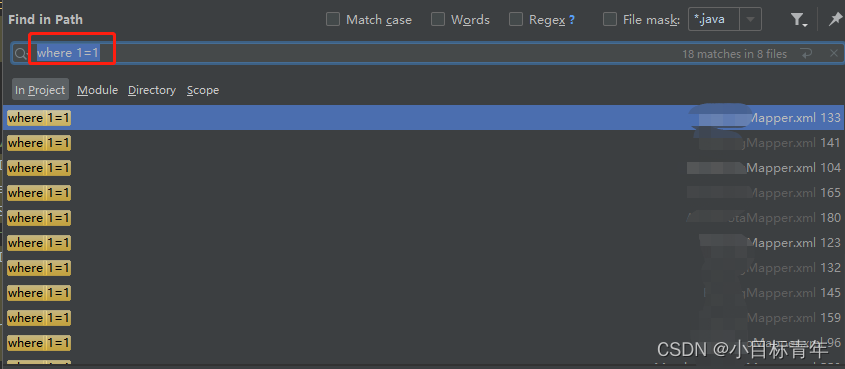那么使用where 1=1 ，会怎么样么 。

首先，先辟谣。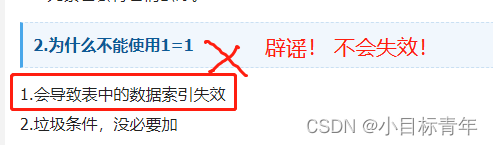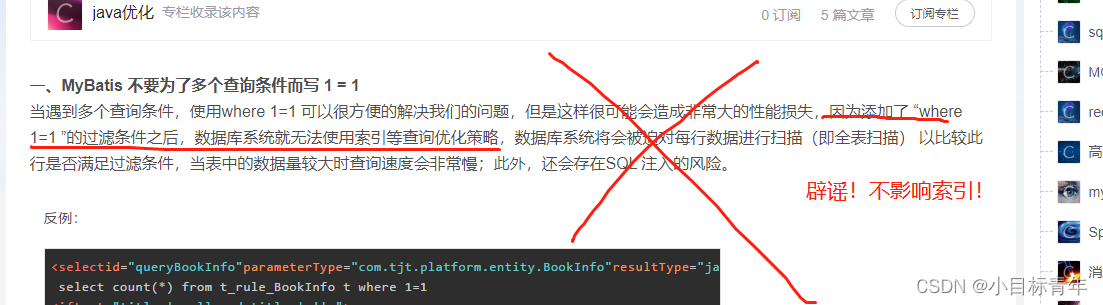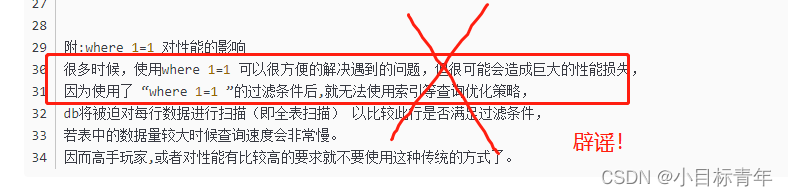where 1=1 不会导致索引失效 ！
where 1=1 不会导致索引失效 ！
where 1=1 不会导致索引失效 ！

客观看待 ：

为什么会去写这个where 1=1 ？

## WHERE 1=1

示例：

<select id="queryList" resultMap="BaseResultMap">
select
<include refid="Base_Column_List"/>
from user_info
where 1=1

<if test="type !=null">
and TYPE = #{type}
</if>
</select>

可能很多人看到这，已经知道，其实mybatis有where 标签是专门用于这种场景的。

而且绝大多数人都知道where标签，只是还是习惯使用 where 1=1 .

其实就是两个点 ：

1. 复制粘贴，随便哪个查询都是if 标签， 复制改一改， 为了不报错，加上 where 1=1 ，完事。
2. 知道mysql 会将接收到的sql 指令做优化，这种where 1=1 是会被优化掉的，所以不影响。

不影响索引命中：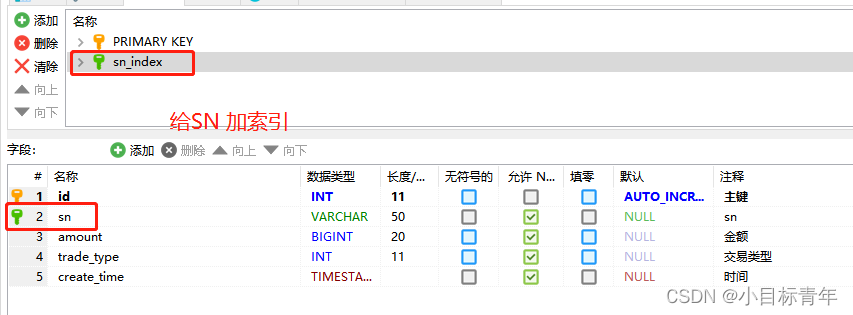不使用 where 1=1 ，能够命中索引：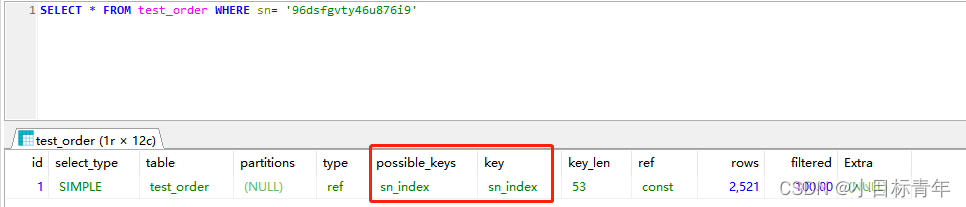使用 where 1=1 ，一样命中索引：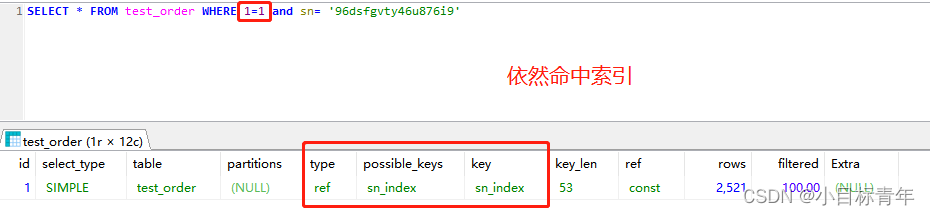所以再说一次 ：  where 1=1 不会导致索引失效 ！

## Mybatis where标签

示例：

<select id="queryList" resultMap="BaseResultMap">
select
<include refid="Base_Column_List"/>
from user_info
<where>
<if test="type !=null">
and TYPE = #{type}
</if>
</where>
</select>

可以看到使用where标签的话，第一个感觉就是，代码顺畅一点，但是多了一个where标签的使用知识需要知道。

可以看到，我的示例，上面 where标签  里面 的第一个if标签里面 有写 and 。

这个是没影响的，mybatis也会帮我们做优化，第一个 and这种东西没关系的。

因为多个if标签同时存在的时候，只要保证触发了的标签  两两之间是用 and 或者 or这些连接起来就行， 第一个会自动优化掉。当然这是mybatis帮我们做的事情。

我怎么知道？看源码看的 ：
mybatis where标签的源码：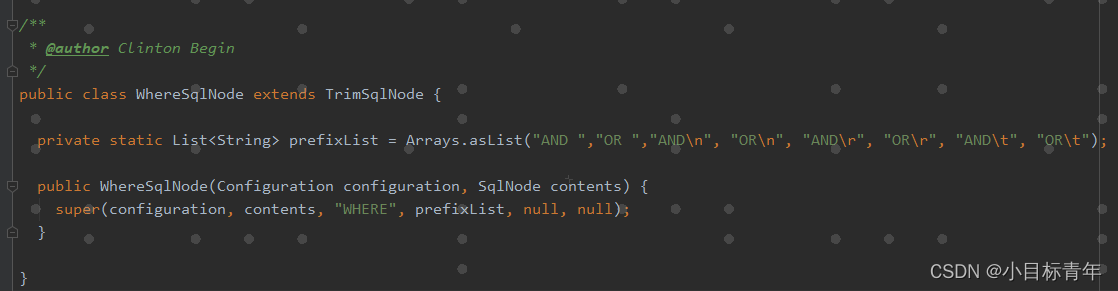可以看到代码里面 where标签 只是定义了一下 所谓的前缀list，实际上还是用的super。

继承的父类，其实就是 trim 标签。
所以我们使用where标签写的示例，也可以改成使用trim标签 ：

<select id="queryList" resultMap="BaseResultMap">
select
<include refid="Base_Column_List"/>
from user_info
<trim prefix="WHERE" prefixOverrides="AND | OR" >
<if test="type !=null">
and TYPE = #{type}
</if>
</trim>

</select>

trim标签 ，也可以瞄一眼源码：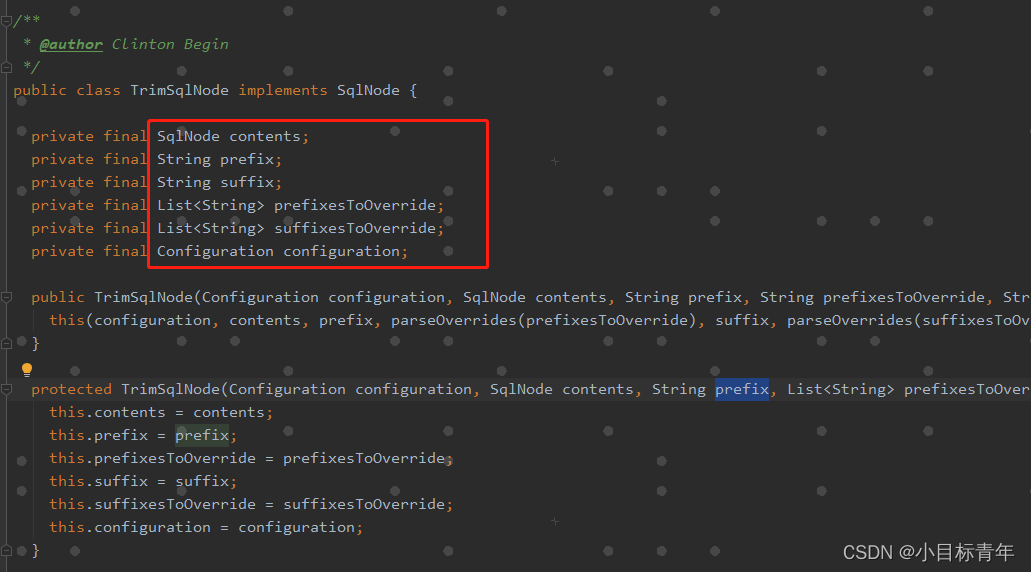prefix：给trim标签内sql语句加上前缀
suffix：给trim标签内sql语句加上后缀
prefixOverrides：去除多余的前缀内容，如：prefixOverrides=“AND”，去除trim标签内sql语句多余的前缀"AND"
suffixOverrides：去除多余的后缀内容，如：suffixOverrides="，"，去除trim标签内sql语句多余的后缀"，"

那么mybatis怎么去处理这个where标签（包括任何其他标签）的呢？ 其实mybatis源码上有相关的测试函数，也可以看的是怎么给我们如何解析where标签，最后拼接成sql的。

感兴趣的可以自己看看（如果你自己有想法，其实可以自己定义自己的一套规则，反正最终是解析拼接出sql语句。）：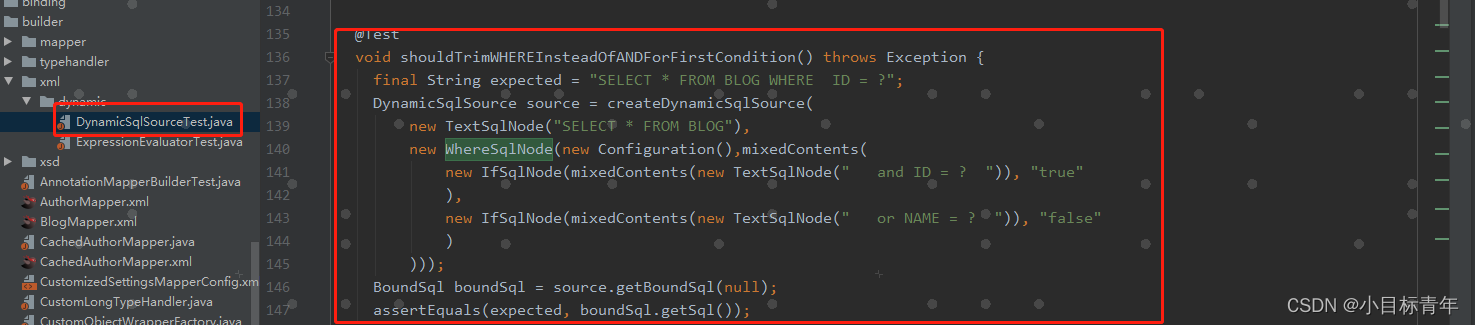## 值得思考的问题

扯远了，那么回到正题， 客观看待使用 where 1=1 .

上面列了一些where标签的源码，为什么我要列这些。

其实就是为了引出一个值得思考斟酌的问题？

使用 where 1=1 ，mysql底层会帮我们优化， 不影响索引。

使用where标签， 则需要 代码层面 帮我们解析。

那么这样来说，是不是使用where标签，其实也会存在性能问题，毕竟要走代码解析？

那么是选择在java代码应用层的解析还是选择 mysql底层的优化呢？

这个问题留给大家思考。

展开全文java mybatis
• ## 不要再用where1=1了，有更好的写法

千次阅读 热门讨论 2022-03-01 07:55:39
背景 刚入行的同学，看到在SQL语句中出现where 1 = 1...如果要问在SQL语句的where条件中多加1=1目的是什么，很简单：使得where条件语句永远为真。本质上就是虽然加了where条件，但实际上永远为真，也就相当于没有加任何

## 背景

刚入行的同学，看到在SQL语句中出现where 1 = 1这样的条件可能会有所困惑，而长时间这样使用的朋友可能又习以为常。

那么，你是否还记得当初为什么要这样写？是否有性能问题？有没有更好的写法？

今天这篇文章，带大家从头到尾梳理一下where 1 = 1的使用及改进，或许你能从中得到更多意想不到的收获。

## where 1=1的作用

如果要问在SQL语句的where条件中多加1=1目的是什么，很简单：使得where条件语句永远为真。本质上就是虽然加了where条件，但实际上永远为真，也就相当于没有加任何约束条件。

使用该语句的场景主要是：动态构建SQL语句

String sql  =  "select * from t_user  where 1=1 ";
if(!b.equals("")){
sql += "and  b='"+b+"'";
}


在上述语句拼写时，使用where 1=1，当b不等于空时，可以直接拼接“and”语句，而不会导致语法错误。如果没有where 1=1，原来的SQL语句就变成（假设b传入了"abc"）：

"select * from t_user  where and  b= 'abc' ";


很明显，上述SQL语句会有语法错误。所以，之所以添加1=1，就是为了方便SQL拼接。

从另外一个角度来讲，不仅仅1=1可以这样使用，像：1<>22>1'a'='a'等表达式，只要结果为true，都可以如此使用。

## where 1<>1

上面讲了where 1=1的来历及使用，那么你是否使用过where 1<>1的形式呢？

你还别说，where 1<>1也是有使用场景的，比如：只获取表结构而不取数据。

create table t_temp as select * from t_user  where 1<>1


上述语句，创建了一个与t_user表结构一样但没有任何数据的新表t_temp。

当然，除了表结构，其他的结构也可以如此使用。

## where 1=1的性能问题

有人说，使用where 1=1可能会有性能问题，咱们直接来实验一下。

mysql 8.0.18，t_user表，id_no字段有索引：

explain select * from t_user where id_no = 'Tom25';
explain select * from t_user where 1=1 and id_no = 'Tom25';


执行上述两行SQL语句，explain结果都是如下：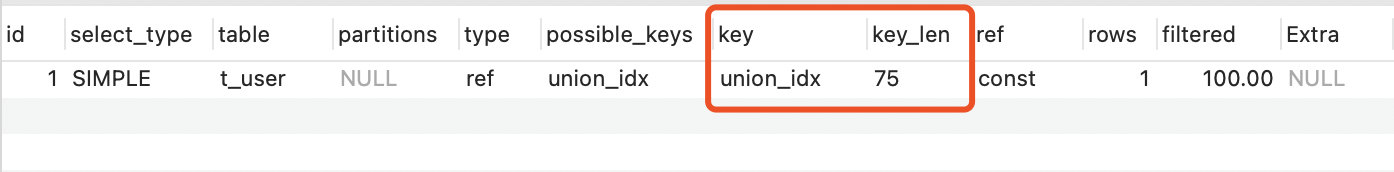也就是说，1=1这样条件，并不影响索引和性能，从explain结果上可以看出两者并无本质区别。

之所以不同的SQL语句，呈现了相同的结果，这是因为被Mysql优化了。Mysql在处理指令时，会对1=1这类无效的条件进行优化处理。这个与Java的编译器有些像，很多无效的判断或语句，在编译成字节码时，编译器会进行优化处理。

## where 1=1的改进

虽然说1=1会被优化器优化掉，但优化操作本身还是会消耗MySQL的性能的，如果能够从根本上避免这种情况的出现，那不就更好了。

以Mybatis为例，在使用where 1=1时，通常会是如下写法：

<select id="queryUser" parameterType="com.choupangxia.entity.User" resultType="java.lang.Integer">
select count(id) from t_user u where 1=1
</if>
<if test="userNo !=null and userNo !='' ">
AND u.user_no = #{userNo}
</if>
</select>


这里where 1=1的作用同上。但如果你更进一步去了解Mybatis的语法及标签，可以使用<where>标签来代替where 1=1

<select id="queryUser" parameterType="com.choupangxia.entity.User" resultType="java.lang.Integer">
select count(id) from t_user u
<where>
</if>
<if test="userNo !=null and userNo !='' ">
AND u.user_no = #{userNo}
</if>
</where>
</select>


这样，在查询数据比较大的情况下，可减少MySQL为了优化1=1这样的条件而损失的性能。

## 小结

本文我们从习以为常的where 1=1使用聊起，聊了它的使用场景、MySQL对其优化、以及延伸出来的where 1<>1的使用，同时基于常见的Mybatis框架，如何进一步改进。

其实，写这篇文章想传达的一个思想就是：再小，再习以为常的事物，如果你去思考、研究都会学到很多相关的知识点，也都可以对其进一步优化。

曾经有一个技术大佬说过：再简单的一段代码，如果将访问量扩大100倍、1000倍，都可以会出现问题。不要停止思考和学习！

博主简介：《SpringBoot技术内幕》技术图书作者，酷爱钻研技术，写技术干货文章。

公众号：「程序新视界」，博主的公众号，欢迎关注~

技术交流：请联系博主微信号：zhuan2quan

展开全文sql MySQL mysql优化
• mybatis where 1=1导致索引失效问题探究 在mybatis中常用到if标签判断where子句后的条件，为防止首字段为空导致sql报错，我之前 在where后加了1=1来处理该问题。 后来又用了mybatis的where标签，并自己做过测试...

# mybatis where 1=1导致索引失效问题探究

在mybatis中常用到if标签判断where子句后的条件，为防止首字段为空导致sql报错，我之前 在where后加了1=1来处理该问题。

后来又用了mybatis的where标签，并自己做过测试，where标签会判断优化处理and/or开头的条件。所以直接解决了那个问题。

那么1=1方式有什么不好的呢。会导致索引失效吗？

其中id_card为唯一索引，所以命中了索引。再试试。

EXPLAIN SELECT * FROM USER WHERE 1=1 AND id_card='622772199303134433' AND age=20 ;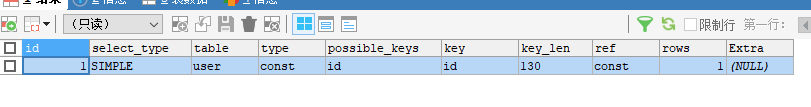为了测试 sex+age设为联合唯一索引

EXPLAIN SELECT * FROM USER WHERE sex=1 AND age=20 ;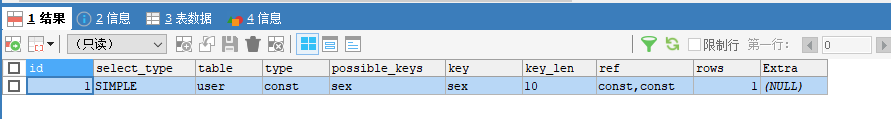EXPLAIN SELECT * FROM user WHERE 1=1 AND sex=1 AND age=20 ;依然命中了索引

那么1=1到底会不会让索引失效呢？

where 1=1不会影响性能。sql优化器已经处理过了。所以两种方式都可以。


<!-- 查询 不触发索引-->

<select id="findUser" resultType="com.cnzz.mybatis.PO.UserPO">

SELECT * FROM user WHERE 1=1

<if test="idCard !=null and idCard!=''">

and id_card=#{idCard}

</if>

<if test="name !=null and name!=''">

and name=#{name}

</if>

<if test="age !=null">

and age=#{age}

</if>

<if test="sex !=null">

and sex=#{sex}

</if>

;

</select>

<!-- 查询 首部字段为空，是否会报错-->

<select id="findUserNew" resultType="com.cnzz.mybatis.PO.UserPO">

SELECT * FROM user

<where>

<if test="idCard !=null and idCard!=''">

and id_card=#{idCard}

</if>

<if test="name !=null and name!=''">

and name=#{name}

</if>

<if test="age !=null">

and age=#{age}

</if>

<if test="sex !=null">

and sex=#{sex}

</if>

</where>

;

</select>

展开全文• 情景分析 工作当中，动态sql经常会用到，当遇到多个查询条件时，我们可能会写出类似下面这样的sql <... select * from user where <if test="username != null"> username=#{usernmysql sql mybatis
• EXPLAIN SELECT * FROM user_point_detail_info WHERE 1=1 AND deleted = FALSE AND app_id = 2010001 AND point > 10 AND add_time BETWEEN "2021-03-12 17:00:00" AND "2021-03-12 17:51:00" ORDER BY user_id ...sql mysql
• ## SQL中where1 = 1的用处

千次阅读 2019-04-13 14:40:38
0. 前言 在刚进公司实习时，看见数据分析的小姐姐写sql时加了一句where 1 = 1，我就很好奇这是啥操作，不是多此一举么，后面...sql语句中where 1=1的作用 sql where 1=1和 0=1 的作用 where 1 = 1，--永远为真 whe...
• ## where1=1和where1=0

千次阅读 2018-07-05 23:30:19
1.where 1=1 select * from where 1=1 and if(a.id=2,'true','false')加where 1=1不管if有没有满足where后总是有条件的。select * from where if(a.id=2,'true','false')不加where 1=1,如果if条件均不满足则where后...hive
• 因为查询条件的不确定，用到了where 1=1这样的权宜之计，使用WHERE 1=1 之后，就不能使用索引了且会遍历全表，显然在数据量比较大的情况下这是不能接受的 解决方法之一：在返回自定义sql之前检查sql，将不需要的...sql优化 mysql mybatis
• where 1=1;有什么用？在SQL语言中，这个条件始终为True，写这一句话就跟没写一样。select * from table where 1=1与select * from table完全没有区别，其目的就是使 where 的条件永远为true，得到的结果就是未加约束...
• ## 深入理解SQL中where1=1的用处

万次阅读 多人点赞 2017-12-26 16:34:36
前言where 1=1有什么用？在SQL语言中，写这么一句话就跟没写一样。 在我的理解中 SQL中where1=1 有两个用处1、where 的条件为永真select * from table1 where 1=1 与 select * from table1 完全没有区别，甚至...sql select
• ## where1=1有什么用？

万次阅读 多人点赞 2018-01-12 17:18:10
为什么要在sql语句后边加上where 1=1　，对于初次见到的童鞋确实很纳闷。觉得select * from table1 where 1=1 与 select * from table1完全没有区别。其实这种想法是错的。记住本落格尔的名言【存在则合理】~~~不管...
• 当遇到多个查询条件，使用where 1=1 可以很方便的解决我们的问题，但是这样很可能会造成非常大的性能损失，因为添加了 “where 1=1 ”的过滤条件之后，数据库系统就无法使用索引等查询优化策略，数据库系统将会被迫...
• mybatis 千万不要使用 where 1=11.解决方案2.为什么不能使用1=1 1.解决方案 下面是mybatis查询语句，如果我们这次我们将 “state = ‘ACTIVE’” 设置成动态条件，看看会发生什么。 <select id=...mybatis
• ## 为什么要用 where1=1

千次阅读 2019-07-14 19:08:45
这个1=1常用于应用程序根据用户选择项的不同拼凑where条件时用的。 例如：查询用户的信息，where默认为1=1，这样用户即使不选择任何条件，sql查询也不会出错。如果用户选择了姓名，那么where后面追加“and 姓名='...mysql
• 将上文提到的（select * from student where）改为（select * from student where 1=1）就实现了这样的效果。倘若不加任何东西，系统会认为这个 sql 语句就是（select * from student ）。但是注意，如果你拼接了...
• ## MySQL中where1=1真的会影响性能么？

万次阅读 多人点赞 2020-01-17 17:41:33
先说结论，where 1=1不会影响性能，如有错误，请指点一下 trim标记是一个格式化的标记，可以完成set或者是where标记的功能。 2020.1.17，周五，距离春节假期返家仅剩两天，在公司抽空做了下代码代码优化，发现有段...
• （一）where 1=1的用法 在写项目中需要建立一个动态的sql，这时可以使用where 1=1. 实例：  String sql=”select name,age from usertable where”； //这里我们没有加where 1=1  　if(!"".equals(user.getName...
• 我们先来看看这个语句的结果：select * from table where 1=1，其中where 1=1，由于1=1永远是成立的，返回TRUE,条件为真；所以，这条语句，就相当于select * from table，返回查询表中的所有数据。一、不用where 1=...sql
• Mysql中where 1=1 和count(0) 使用小技巧mysql中使用 where 1=1和 1=1 的作用
• 在查询的过程中经常会用到where1=1 在数据量小的时候好像是没有什么影响的，但是到了数据量太大的时候使用where 1=1 的话好像就会降低查询效率，有没有什么好的方法是可以代替这个where 1=1 的能够提高执行效率sql
• 但是使用WHERE 1=1 之后,就不能使用索引了,从MySQL5.7开始,会自动默认取消掉SQL中第一个为true的条件，而且每一条数据都得查询一遍，效率低。 如何解决WHERE 1=1 的问题 (加个flag) boolean isFirst = ture;...sql 优化
• where 后面表示过滤的条件，只有满足条件的记录才被选择，因为条件（1=2）为假， 所以整句话其实就是什么都不选择，比如我要建一个和表a相同结构的表b 但我不要数据就可以： select * into 表b from 表a ...
• 1=1 永真， 1<>1 永假。 select * from sys_voter ...select * from sys_voter t where 1=1 查询全部数据 相当于 select * from sys_voter t ； 那么这句的话有啥作用呢？？ sql语句a : select * from sys_voter t whemybatis sql
• select from SAMPLE where 1=1 AND SAMPLENUMBER = #{samplenumber,jdbcType=DECIMAL} Mybatis 之前拼条件的时候 写法 where 1=1，也可以使用标签 <select id="findActiveBlogL
• 什么时候用到where 1=1 动态构建SQL语句。 如果要问在SQL语句的where条件中多加1=1目的是什么，很简单：使得where条件语句永远为真。本质上就是虽然加了where条件，但实际上永远为真，也就相当于没有加任何约束条件...java mysql
• ## sql where1=1 规范代码

千次阅读 热门讨论 2016-01-19 19:30:31
select * from user where 1=1这两个 句子执行结果是一样一样的。而sql注入就是利用了这个原理 来进行破坏。比如：select * from user where id='1000'如果允许用户输入的话，那么这个句子就成了：select * from ...sql
• where&gt;标签 &lt;select id="findActiveBlogLike" resultType="Blog"&gt; SELECT * FROM BLOG WHERE &lt;if test="state != null"&gt; state = #{...
• 先看一个例子：      SELECT * FROM PERSON WHERE 1=1    AGE > #ageValue#    ]]>       ...1、 SQL中使用了where 1=1 ，很优美的解决了参数中ageValue为空时SQL语法SQL 优化 ORACLE...